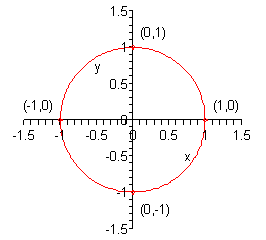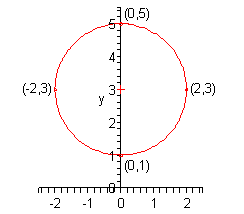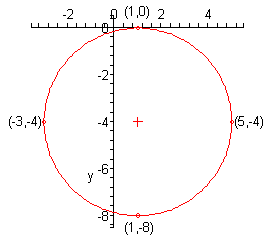Paul's Online Notes
Home / Algebra / Graphing and Functions / Circles
Show Mobile Notice Show All Notes Hide All Notes
Mobile Notice
You appear to be on a device with a "narrow" screen width (i.e. you are probably on a mobile phone). Due to the nature of the mathematics on this site it is best views in landscape mode. If your device is not in landscape mode many of the equations will run off the side of your device (should be able to scroll to see them) and some of the menu items will be cut off due to the narrow screen width.

### Section 3.3 : Circles

In this section we are going to take a quick look at circles. However, before we do that we need to give a quick formula that hopefully you’ll recall seeing at some point in the past.

Given two points $$\left( {{x_1},{y_1}} \right)$$ and $$\left( {{x_2},{y_2}} \right)$$ the distance between them is given by,

$d = \sqrt {{{\left( {{x_2} - {x_1}} \right)}^2} + {{\left( {{y_2} - {y_1}} \right)}^2}}$

So, why did we remind you of this formula? Well, let’s recall just what a circle is. A circle is all the points that are the same distance, $$r$$ – called the radius, from a point, $$\left( {h,k} \right)$$ - called the center. In other words, if $$\left( {x,y} \right)$$ is any point that is on the circle then it has a distance of $$r$$ from the center, $$\left( {h,k} \right)$$.

If we use the distance formula on these two points we would get,

$r = \sqrt {{{\left( {x - h} \right)}^2} + {{\left( {y - k} \right)}^2}}$

Or, if we square both sides we get,

${\left( {x - h} \right)^2} + {\left( {y - k} \right)^2} = {r^2}$

This is the standard form of the equation of a circle with radius $$r$$ and center $$\left( {h,k} \right)$$.

Example 1 Write down the equation of a circle with radius 8 and center $$\left( { - 4,7} \right)$$.
Show Solution

Okay, in this case we have $$r = 8$$, $$h = - 4$$ and $$k = 7$$ so all we need to do is plug them into the standard form of the equation of the circle.

\begin{align*}{\left( {x - \left( { - 4} \right)} \right)^2} + {\left( {y - 7} \right)^2} & = {8^2}\\ {\left( {x + 4} \right)^2} + {\left( {y - 7} \right)^2} & = 64\end{align*}

Do not square out the two terms on the left. Leaving these terms as they are will allow us to quickly identify the equation as that of a circle and to quickly identify the radius and center of the circle.

Graphing circles is a fairly simple process once we know the radius and center. In order to graph a circle all we really need is the right most, left most, top most and bottom most points on the circle. Once we know these it’s easy to sketch in the circle.

Nicely enough for us these points are easy to find. Since these are points on the circle we know that they must be a distance of $$r$$ from the center. Therefore, the points will have the following coordinates.

\begin{align*}& {\mbox{right most point : }}\left( {h + r,k} \right)\\ & {\mbox{left most point : }}\left( {h - r,k} \right)\\ & {\mbox{top most point : }}\left( {h,k + r} \right)\\ & {\mbox{bottom most point : }}\left( {h,k - r} \right)\end{align*}

In other words all we need to do is add $$r$$ on to the $$x$$ coordinate or $$y$$ coordinate of the point to get the right most or top most point respectively and subtract $$r$$ from the $$x$$ coordinate or $$y$$ coordinate to get the left most or bottom most points.

Let’s graph some circles.

Example 2 Determine the center and radius of each of the following circles and sketch the graph of the circle.
1. $${x^2} + {y^2} = 1$$
2. $${x^2} + {\left( {y - 3} \right)^2} = 4$$
3. $${\left( {x - 1} \right)^2} + {\left( {y + 4} \right)^2} = 16$$
Show All Solutions Hide All Solutions
Show Discussion

In all of these all that we really need to do is compare the equation to the standard form and identify the radius and center. Once that is done find the four points talked about above and sketch in the circle.

a $${x^2} + {y^2} = 1$$ Show Solution

In this case it’s just $$x$$ and $$y$$ squared by themselves. The only way that we could have this is to have both $$h$$ and $$k$$ be zero. So, the center and radius is,

${\mbox{center}} = \left( {0,0} \right)\hspace{0.25in}\hspace{0.25in}{\mbox{radius}} = \sqrt 1 = 1$

Don’t forget that the radius is the square root of the number on the other side of the equal sign. Here is a sketch of this circle.A circle centered at the origin with radius 1 (i.e. this circle) is called the unit circle. The unit circle is very useful in a Trigonometry class.

b $${x^2} + {\left( {y - 3} \right)^2} = 4$$ Show Solution

In this part, it looks like the $$x$$ coordinate of the center is zero as with the previous part. However, this time there is something more with the $$y$$ term and so comparing this term to the standard form of the circle we can see that the $$y$$ coordinate of the center must be 3. The center and radius of this circle is then,

${\mbox{center}} = \left( {0,3} \right)\hspace{0.25in}\hspace{0.25in}{\mbox{radius}} = \sqrt 4 = 2$

Here is a sketch of the circle. The center is marked with a red cross in this graph.c $${\left( {x - 1} \right)^2} + {\left( {y + 4} \right)^2} = 16$$ Show Solution

For this part neither of the coordinates of the center are zero. By comparing our equation with the standard form it’s fairly easy to see (hopefully…) that the $$x$$ coordinate of the center is 1. The $$y$$ coordinate isn’t too bad either, but we do need to be a little careful. In this case the term is $${\left( {y + 4} \right)^2}$$ and in the standard form the term is $${\left( {y - k} \right)^2}$$. Note that the signs are different. The only way that this can happen is if $$k$$ is negative. So, the $$y$$ coordinate of the center must be -4.

The center and radius for this circle are,

${\mbox{center}} = \left( {1, - 4} \right)\hspace{0.25in}\hspace{0.25in}{\mbox{radius}} = \sqrt {16} = 4$

Here is a sketch of this circle with the center marked with a red cross.So, we’ve seen how to deal with circles that are already in the standard form. However, not all circles will start out in the standard form. So, let’s take a look at how to put a circle in the standard form.

Example 3 Determine the center and radius of each of the following.
1. $${x^2} + {y^2} + 8x + 7 = 0$$
2. $${x^2} + {y^2} - 3x + 10y - 1 = 0$$
Show All Solutions Hide All Solutions
Show Discussion

Neither of these equations are in standard form and so to determine the center and radius we’ll need to put it into standard form. We actually already know how to do this. Back when we were solving quadratic equations we saw a way to turn a quadratic polynomial into a perfect square. The process was called completing the square.

This is exactly what we want to do here, although in this case we aren’t solving anything and we’re going to have to deal with the fact that we’ve got both $$x$$ and $$y$$ in the equation. Let’s step through the process with the first part.

a $${x^2} + {y^2} + 8x + 7 = 0$$ Show Solution

We’ll go through the process in a step by step fashion with this one.

Step 1 : First get the constant on one side by itself and at the same time group the $$x$$ terms together and the $$y$$ terms together.

${x^2} + 8x + {y^2} = - 7$

In this case there was only one term with a $$y$$ in it and two with $$x$$’s in them.

Step 2 : For each variable with two terms complete the square on those terms.

So, in this case that means that we only need to complete the square on the $$x$$ terms. Recall how this is done. We first take half the coefficient of the $$x$$ and square it.

${\left( {\frac{8}{2}} \right)^2} = {\left( 4 \right)^2} = 16$

We then add this to both sides of the equation.

${x^2} + 8x + 16 + {y^2} = - 7 + 16 = 9$

Now, the first three terms will factor as a perfect square.

${\left( {x + 4} \right)^2} + {y^2} = 9$

Step 3 : This is now the standard form of the equation of a circle and so we can pick the center and radius right off this. They are,

${\mbox{center}} = \left( { - 4,0} \right)\hspace{0.25in}{\mbox{radius}} = \sqrt 9 = 3$

b $${x^2} + {y^2} - 3x + 10y - 1 = 0$$ Show Solution

In this part we’ll go through the process a little quicker. First get terms properly grouped and placed.

$\underbrace {\,\,\,\,\,{x^2} - 3x\,\,\,\,\,}_{{\mbox{complete the square}}} + \underbrace {\,\,\,{y^2} + 10y\,\,\,}_{{\mbox{complete the square}}} = 1$

Now, as noted above we’ll need to complete the square twice here, once for the $$x$$ terms and once for the $$y$$ terms. Let’s first get the numbers that we’ll need to add to both sides.

${\left( { - \frac{3}{2}} \right)^2} = \frac{9}{4}\hspace{0.25in}\hspace{0.25in}\hspace{0.25in}\hspace{0.25in}{\left( {\frac{{10}}{2}} \right)^2} = {\left( 5 \right)^2} = 25$

Now, add these to both sides of the equation.

$\underbrace {{x^2} - 3x + \frac{9}{4}}_{{\mbox{factor this}}} + \underbrace {{y^2} + 10y + 25}_{{\mbox{factor this}}} = 1 + \frac{9}{4} + 25 = \frac{{113}}{4}$

When adding the numbers to both sides make sure and place them properly. This means that we need to put the number from the coefficient of the $$x$$ with the $$x$$ terms and the number from the coefficient of the $$y$$ with the $$y$$ terms. This placement is important since this will be the only way that the quadratics will factor as we need them to factor.

Now, factor the quadratics as show above. This will give the standard form of the equation of the circle.

${\left( {x - \frac{3}{2}} \right)^2} + {\left( {y + 5} \right)^2} = \frac{{113}}{4}$

This looks a little messier than the equations that we’ve seen to this point. However, this is something that will happen on occasion so don’t get excited about it. Here is the center and radius for this circle.

${\mbox{center}} = \left( {\frac{3}{2}, - 5} \right)\hspace{0.25in}\hspace{0.25in}{\mbox{radius}} = \sqrt {\frac{{113}}{4}} = \frac{{\sqrt {113} }}{2}$

Do not get excited about the messy radius or fractions in the center coordinates.